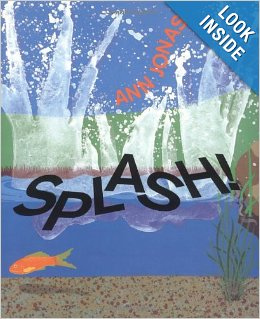# Two Digit Addition and Subtraction Review

5 teachers like this lesson
Print Lesson

## Objective

SWBAT add and subtract within 100; SWBAT practice facts within 20.

#### Big Idea

Lets put it all together! After learning about both single digit and two digit addition and subtraction, this lesson puts all problems together to allow student to practice addition and subtraction strategies.

## Activating Strategy

10 minutes

I like to start this lesson by reading the book Splash, by Ann Jonas.I like to read this book because it has multiple opportunities for students to practice addition and subtraction.  Before reading, I give students 12 connecting cubes, or counters.

While reading the story, I have the student’s model with their manipulatives to demonstrate addition and subtraction with the story.

The standard NBT.C4 is a standard with many objectives, including using concrete models or drawings and strategies based on place value, properties of operations, and/or the relationship between addition and subtraction.  This lesson provides the students with the opportunity to practice strategies to solve various addition and subtraction problems.

## Teaching Strategies

15 minutes

To access prior knowledge, I write the numbers 15, 50, and 51 on the board or chart paper (also available as a PPT Addition and subtraction review in the resources section). I then guide the discussion:

• How can you show tens and ones in each number? ( I can show 15 with 1 ten and 5 ones; I can show 50 with 5 tens; I can show 51 with 5 tens and 1 one.)

• How are 15 and 51 alike? (They are both two-digit numbers. They have the same digits.)

• How are 15 and 51 different? (15 has 1 ten and 5 ones. 51 has 5 tens and 1 one.)

Then I write the following problem on the board/chart paper:

The class collects paper bags for an art project.  Ron brings in 7 more bags than Ben.  Ben brings in 30 bags.  How many bags does Ron bring?

To help guide students through this problem, I remind them of the strategy to think through what information do they need to find, what do they know that can help solve the problem, and how can we draw a picture to help solve the problem.

I give the students time to solve the problem using counters, connecting cubes, or by drawing a quick picture.  I allow them time to decide on their own strategy.  I then have volunteers come to the front of the room and I ask:

• How did you solve the problem?

I give them time to explain and demonstrate their strategy to the class.

I then write the following problems on the board/chart paper:

5 + 9 = _____

50 – 30= _____

51 + 21 = _____

I again call on volunteers to come and demonstrate their strategy for solving the problems.

When all three problems are finished, I remind students of our strategies that we have learned for adding and subtracting:

• counting on
• counting back
• drawing pictures
• using manipulatives

Learning a variety of approaches empowers children. Since children have many different modalities, teaching a variety of strategies and showing different models for concepts and operations helps all children find a way that works for them. It also gives children alternative methods of problem solving when they are having trouble finding a solution. For example, knowing several ways to add 2 two-digit numbers gives children the ability to choose a method that can be used to meet the needs of a particular situation.  By allowing students to determine their own strategy, they are modeling with math (MP4) by using a variety of methods to solve problems.

## Independent Practice

30 minutes

For the independent practice portion of this lesson, I hand out Addition and subtraction review_worksheet that contains one digit and two digit addition and subtraction problems.  This allows for students to continue to work on their fluency and to practice the multiple strategies we have practiced.

For struggling students, I allow them to use counters, base ten blocks or other manipulative that they need to support their mathematical practice.

## Closing/Summarizing

5 minutes

To close out the lesson, I allow students to pick a problem from their worksheet, and write it on a post-it note. We then mix, pair, share to close out the lesson.

To mix, pair, share - each student walks around the room with their problem until I say, "stop" - when I say stop, I then begin to count to 5.  By the time I get to 5, the expectation is that each student has a partner.  The partners then exchange their post it note, and they solve each other's math problems.  This allows for an opportunity for all levels of students to work together and to problem solve together.  Once they have solved each others math problems they get their original post it note back and we mix again.